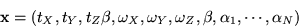Next: Biased Measurements Up: The Proposed Nonlinear Recursive Previous: The Issue of Scale

## The EKF Implementation

Using the representations discussed thus far, our composite state vector consists of 7+N parameters--6 for motion, 1 for camera geometry, and N for structure--where N is the number of features tracked:(19)

The vector x is the state vector used in a standard EKF implementation, where the measurement vector contains the image locations of all the tracked features in a new frame. As described earlier, an additional quaternion is required for maintaining a description of the global rotation external to the EKF.

The dynamics model in the EKF can be chosen trivially as an identity transform plus noise, unless additional prior information on dynamics is available. The measurement equation is simply obtained by combining Equations 1215, and 14. The RHS (u,v) in Equation 14 is the defining image location of the feature in its initial frame, and the LHS (u,v) in Equation 12 is the measurement.

The final implementation of the EKF is straightforward (standard references include [10,12,21]), with the only additional computation being the quaternion maintenance. Computationally, the filter requires inverting a 2Nx2N matrix (i.e. the size of the measurement vector) [12,21], which is not a large task for the typical number of features on a single object. Since all parameters are overdetermined with 7 or more points, N rarely needs to be more than 15 or 20 for good results, yielding filter steps which can be computed in real-time on modern workstations.Next: Biased Measurements Up: The Proposed Nonlinear Recursive Previous: The Issue of Scale

1999-05-17